﻿ How Choosing Precisely the Secondary Length of an Electromagnetic Catapult Could Prevent VibrationsPublications are Open
Access in this journal
Article Versions
Export Article
• Normal Style
• MLA Style
• APA Style
• Chicago Style
Research Article
Open Access Peer-reviewed

### How Choosing Precisely the Secondary Length of an Electromagnetic Catapult Could Prevent Vibrations

Jean-Pierre Pascal
American Journal of Electrical and Electronic Engineering. 2019, 7(2), 26-33. DOI: 10.12691/ajeee-7-2-1
Received April 25, 2019; Revised June 09, 2019; Accepted June 21, 2019

### Abstract

Dealing with electromagnetic catapults, using a simplified numerical approach discretizing the secondary into small parallelepipeds, we demonstrate that short secondary linear induction motors usually produce superimposed periodic force modulations (large ripple). The paper begins with analyzing force modulations in the simple case of an aluminum sheet moving at constant speed inside a stationary sinusoidal magnetic field. The second part extends the analysis to the motor case when both the magnetic field and the sheet are moving at different speeds with an algorithm controlling accelerations through the motor slip. The third part presents an application of the calculation method simulating vibrations of a suspended mass elastically linked to a launching aircraft. The paper concludes that the linear motor thrust is modulated at a variable frequency which depends upon the motor control strategy. The frequency of this modulation is the ratio of the speed difference, between the field velocity minus the motor velocity, divided by the motor pole pitch. The thrust modulation could induce strong vibrations on heavy parts, such as fuel tanks, suspended onto aircraft wings, when meeting their resonance frequency. It is demonstrated how this modulation is avoided completely when the secondary motor length is an exact multiple of the stator pole pitch.

### 1. Introduction

During the last century, aircraft carriers have been constructed, mainly in America, all of which used steam catapults for launching aircrafts. Steam catapults present several drawbacks, mainly the lack of adaptability and large maintenance requirements.

In the middle of the century, using Linear Induction Motors (LIM) as catapults has been attempted in America with the Westinghouse ‘Electropult’ system, in 1945 1. However, power electronics was not available yet and the project had to be abandoned.

Later, in France, on the basis of a new linear motor structure 2, 3, a study was made for an electromagnetic catapult intended for the Charles de Gaulle carrier. But, in the frame of NATO organization, for compatibility reasons, the steam catapult was chosen at the end of the day.

At the end of the century, the project of an electromagnetic catapult was launched again in the US using a linear motor with permanent magnets 4. It was not further developed.

From 2004, General Atomics company, developed for the US Navy another electromagnetic catapult, also known as EMALS (Electro-Magnetic Aircraft Launch System), based on an asynchronous induction linear motor with a long stator of constant pole pitch and a moving short secondary in the shape of a rectangular aluminum sheet. This project succeeded in testing a full size catapult, launching heavy aircrafts from the Lakehurst facility. A first operational electromagnetic catapult was installed on the first of type carrier G. Ford which launches aircrafts at sea since July 2017.

However, since 2014, press and Pentagon reports have been disclosing that dangerous vibrations occurred on loaded wings of aircraft fighters when using this EMALS catapult 5.

Academic literature 6, 7, 8, 9 thoroughly explains how to build models to calculate the characteristics of electrical induction motors, either using the equivalent circuit model or the Circle Diagram. Applying this knowledge to linear motors allows calculating their main characteristics. But calculation models applied to linear induction motors, even though taking end effects into account, do not allow simulating the origin of the reported vibrations 10, 11.

Reference 12 deals with the peak to peak ripple in propulsive force of linear induction machines and finds its dependence on the length of the primary. The dominant frequency of the ripple is found twice the supply frequency.

Present paper explains how a simplified numerical approach succeeds in calculating motor forces with superimposed periodic force modulations (large ripple) of which the frequency does not depend only on the supply frequency, but also on the pole pitch and the motor speed. These modulations are able to excite vibrations of suspended masses onboard aircrafts such as wing mounted fuel tanks.

The paper begins with analyzing a simple electromagnetic brake arrangement and points physically to the origin of force modulations in the simple case of an aluminum sheet moving at constant speed inside a fixed sinusoidal magnetic periodic field. Similar force modulations are found even though the periodic field is not sinusoidal.

The second part, using the results of the brake simulation, extends the analysis to the motor case when both the magnetic field and the sheet are moving at different speeds whilst an algorithm controls speeds using the motor slip.

The third part presents an application of the calculation method resulting in strong vibrations of a suspended mass linked to the launched aircraft.

The paper concludes that the short stator linear induction motor thrust is always modulated at a frequency which depends upon the motor slip and the stator pole pitch. This makes it more or less unpredictable because complex control algorithms of induction motors are intended towards improving efficiency. The frequency of this modulation is the ratio of the speed difference, between the field velocity minus the motor velocity, divided by the motor pole pitch. If the thrust modulation frequency meets the resonance of heavy parts, such as fuel tanks, suspended onto the aircraft wings it can induce strong vibrations.

The conclusion suggests this analysis as a possible explanation for the EMALS vibrations. It insists on stating clearly that this unfavorable modulation could be avoided only if the secondary motor length would be an exact multiple of the stator pole pitch.

### 2. Metallic Sheet Moving into a Periodic Magnetic Flux (Figure 1)

2.1. Model Description and Equations

To begin with understanding the ripple problem it is easy to deal at first with a rectangular plate moving inside a stationary periodic magnetic field, this makes a brake arrangement. In the next section, we will increase the problem complexity to the motor case by also moving the magnetic flux so that there will be several moving space references.

Figure 1 presents a rectangular aluminum sheet moving at constant velocity, Vx, in a fixed reference frame 0XYZ where exists a stationary magnetic flux of which the vector density B has only one component By(x) normal to the sheet. By(x) is constant along 0Z and is periodic along 0X: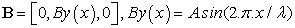(1)

By(0) = 0 and By(x) = 0, if x < 0.

The field wave length,λ, is the double of the inductor pole pitch: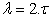(2)

The sheet characteristics are in Table 1:

Top and bottom of the sheet have short-circuit bars of negligible resistance.

Two cases for the length Ls: either any value in the general case, or a whole number ratio, N, to the pole pitch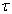as a specific case: Ls/= N.

• Figure 1. A metallic Sheet moving at speed Vx into a magnetic Flux of periodic density By(x)

We will use only physical laws of electromagnetism such as described in ref 13. Displacement currents are ignored.

Let us consider, Figure 1, a small element parallelepiped of length dx in the sheet as an isolated conductor of height Hs and width Ws. For a current di flowing in 0Z direction, this dx part has a resistance dR: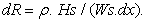(3)

Due to Faraday’s law and speed Vx, there will be an EMF, Uz, developed across this parallelepiped: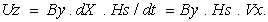(4)

Assuming that Uz is periodic and that the induced currents can close through adjacent poles due to short–circuit bars, owing to Ohm’s law, the current di flowing in the dx part will be :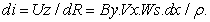(5)

Due to Lorentz force law, there will be a force dF acting on the current di which is submitted to the flux density By: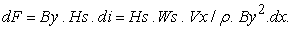(6)

Thus, to calculate the force F(x) acting on the metallic sheet of which the front end is located at abscissa x, we have to sum up dF along the length Ls of the sheet; here F(x) is a braking force opposite to Vx: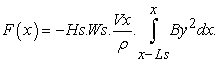(7)
2.2. General Case: Ratio K=Ls/Takes any Value but not a Whole Number (Figure 2 - Figure 3)

Let’s take the example K = 2.5,= 0.5 m, Ls = 1.25 m, Vx = 72 m/s, A = 1.058 Tesla, in Eq. (1), for x = 6.25 m, we calculate easily: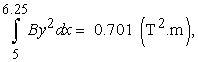and, applying Eq. (7):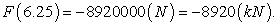(8)

But, the Figure 2, which displays the function By2 together with its sum along the sheet, for two locations, A: x = 6.25 m and B: x = 7.875 m, shows that, during the sheet displacement along 0X, the integral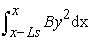is not constant, for example: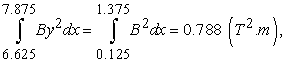with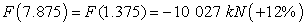(9)

and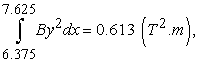with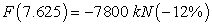(10)

And finally, we have a rule (obvious for sinus shapes with=2π): if N is a whole number, it results :

If x = N .2 , then F(x) = -8 920 kN, as in Eq. (8)

If x = N .-4 , then F(x) = - 10 027 kN as in Eq. (9)

If x = N+4 , then F(x) = -7 800 kN as in Eq. (10)

Thus, when calculating continuously the force F(x) during the displacement of the sheet, using equation (7), as shown in Figure 3, we get a modulated force F with a ripple wavelength equal to the pole pitchof the stationary magnetic field.

The Figure 3 displays the force F(x) acting on the sheet, as a function of the location x of its front end. x = 0 when the metallic sheet front enters at constant speed, Vx, into the space where the magnetic flux is present. The bottom line shows the phase shift with the function (By2), amplified 1000 times.

F(x) sign in Figure 3 has been changed from (-) to (+).

F(x) increases from 0 until the sheet is completely covered by the field, then, at the constant speed Vx, the average force is constant with a superimposed modulation (large ripple) of which the wavelength is equal to the pole pitch of the inducing flux.

2.3. Specific Case: Ratio Ls/= N , a Whole Number (Figure 4 - Figure 5)

Let’s calculate the example N = 2,= 0.5 m, Ls = 1.0 m, Vx = 72 m/s, A = 1.332 Tesla, in Eq. (1).

We calculate easily: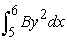= 0.888 (T2.m), and, applying Eq. (7):• Figure 2. K = 2.5: How the Sum of By2.dx along the sheet length depends on the sheet location
• Figure 3. K = 2.5: The Braking force F retarding the moving sheet is modulated at a wavelength( F = 8920 kN +- 12%)
• Figure 4. K = N = 2: How the Sum of B2.dx along the sheet length does not depend on the sheet location
• Figure 5. K = N = 2 : Once totally into the flux, the Braking force F retarding the moving sheet is constant
• Figure 6. Aircraft Launching Simulation : Tank Mt is towed behind Aircraft Ma driven by the motor thrust Fm and Jet Fj

We can easily show, as in examples of Figure 4, that the sum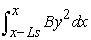is constant and does not depend on x. Situation A is for x = 6 m and situation B is for x = 7.125 m. Consequently, the Figure 5 shows that the force F(x) does not depend on the sheet situation as soon as it has completely entered into the space where the magnetic flux is stationary. Thus, for avoiding the force modulation, in this braking situation of a metallic sheet moving at constant speed inside a periodic stationary magnetic flux, we have found a solution which is general: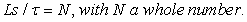(11)

This rule is quite general, whatever the stator pole pitchor the Length Ls of the short secondary, the braking force is constant, without superimposed modulations, as soon as the ratio Ls/is a whole number.

In return, superimposed periodic modulations will be added to the braking force for all situations when the ratio Ls/is not a whole number (see Figure 3).

### 3. Linear Motor Case: the Exciting Periodic Magnetic Flux is Moving

3.1. Aircraft Catapult Case (Figure 6)

The linear induction motor case is similar to the previous case, with a metallic sheet moving inside a magnetic periodic flux, like in Figure 1. But now, both the magnetic field and the sheet are moving at different speeds along 0X with some algorithm controlling speeds and motor slip using a feedback control 14.

This linear motor theory follows general induction motors analyzes such as found in books 6, 7. Let’s just fix at first some notations. As all velocities are along 0X axis, we will omit subscript x. We will use ‘s’ for parameters related to the secondary moving sheet, ‘f’ for parameters of the moving excitation magnetic field and ‘r’ for parameters relative to both systems:

- Vs = velocity of the sheet, often said motor speed, as seen in fixed frame

- Vf = velocity of the magnetic excitation field, as seen in fixed frame

- Vr = relative velocity = Vf – Vs, it is the field velocity seen inside the moving sheet reference frame

- s = motor slip defined as :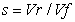(12)

- Fra = frequency of the generator

- Frm = frequency of the unfavorable motor force modulation

Using the previous braking section 2 analysis it is easy to develop a similar method calculating the thrust force of a linear induction motor with a short moving secondary in the shape of a metallic sheet. The motor speed Vx must be replaced by the relative velocity Vr in the electromagnetic equation (4).

We have developed the calculation program in order to use it for the aircraft catapult case.

The Catapult problem is, using the thrust Fm of the linear motor, to launch an aircraft of mass, Mav, up to a speed, Vs, on a track of limited length, Lt. In addition, the Jet Engine is always on at full throttle with a thrust Fj.

Once the force Fm reaches its target value, it should not be modulated.

Measured excitation of resonance frequencies during aircrafts launching could be related to the superimposed modulation of the motor force. And that’s why present study aims at better understanding and possibly correcting these phenomena by modeling them as simply as possible.

In examples, we will deal with Table 2 data:

3.2. Organization of the Calculation Program (Fortran Code)

Physical electromagnetic equations used in the code are the same as above equations (1) to (7). Figure 1 notations will be used. All equations will be solved using numerical digitalization of the space, using small increments dx, and of the time, using small increments dt.

At first a subroutine of the code calculates speed and force targets, functions of time and space, for the case, using classical dynamical equations.

Then the code is organized in two intertwined numerical loops:

3.2.1. Main Loop or ‘Mechanical Loop’

It digitizes the time from 0 to the end of the launching. It uses the outputs of the second loop, namely the motor force Fm(t-dt), to calculate the dynamics of the system, solving Newton’s equations.

This part also uses a control algorithm which was developed to adjust the motor slip, s, to get the required speed and force such as to follow previous calculated targets. This algorithm aims at the best possible motor efficiency taking into account the available flux density. It uses a double PID strategy controlling both the field frequency and the power voltage.

From s and Vs, using Eq. (12), it calculates Vf and Vr

Then, at each time t, it calls the second loop to calculate the force Fm(t).

3.2.2. Secondary Loop or ‘Electromagnetic Loop’

It starts from the first loop data at time t:

Xs(t) = location of the sheet in the fixed reference frame

Vs(t) = speed of the sheet in the fixed reference frame

Vf(t) = speed of the field in the fixed reference frame

Wr(t) = frequency of the excitation as seen in the sheet frame

This secondary loop digitizes the length of the sheet by small dx increments from one end to the other through Nx steps, dx = Ls/Nx. The current index, n, starts at 1 up to Nx. At each step n, it calculates :

The value of the flux density Bn , Eq. (1)

The FEM, Un , Eq. (4)

The current, din , Eq. (5)

The force dFn , Eq. (6)

At the end of this loop, its calculates the sum: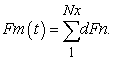Then it returns to the main loop. The calculation stops when either the target speed is reached or the available track distance is exceeded or the specified time is exceeded.

3.3. Calculation Results – Examples of Different Ls/Ratios

In these cases (launching data of Table 2) the aircraft mass is considered as one solid rigidly attached to the motor secondary: Aircraft and secondary motor masses move together as one same solid. Motor data are in Table 3.

The wavelength of the motor force modulations is, as in the brake case, linked to the motor pole pitch,, but its frequency, Frm, is linked to the relative velocity, Vr, instead of the motor speed, Vx, :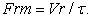(13)

With Vr = Vf-Vs, Eq. (12), and Vf = 2.Fra, we get :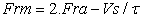(14)

Fra = frequency of stator current

Frm = frequency of the thrust modulation

Vs = velocity of the sheet (motor speed).

Thus, once the target speed, Vs(t), is calculated for a launching, the frequency, Frm, of the motor thrust modulation will depend on the frequency of the traveling magnetic field which is the frequency, Fra, of the primary motor current. Fra is governed by the required motor slip, s, which itself depends on the chosen motor control strategy which is normally dedicated to improving efficiency. This means that the ripple frequency, Frm, varies during one launching and depends not only on the motor pole pitch but also on the motor control strategy and feedback gains. This makes it difficult to avoid resonances of suspended devices either having definite frequencies or depending upon variable parameters such as the amount of fuel inside tanks.

3.3.1. Case 1: There are no Fuel Tanks, K = 12.5 (Figure 7 – Figure 8)

The average motor thrust is 920 kN after a smooth increase during 0.6 seconds (Figure 7). The superimposed modulation is about 5% . The motor-aircraft speed reaches its target of 72 m/s after 2.6 seconds. Ls/= 12.5

The relative speed Vr (Figure 8) reaches 4.7 m/s and the value 4.25 m/s can be accepted for calculating an average ripple frequency Frm= 4.25/0.5 = 8.5 Hz between times t = 0.6 s and t = 2 s (Eq. 13).

• Figure 7. Case 1: K = 12.5, the Motor Thrust is modulated
• Figure 8. Case 1: K = 12.5, the relative speed is modulated

3.3.2. Case 2: There are no fuel tanks, K =12 (Figure 9)

The Figure 9 shows the same force as in Figure 7 case 1, but with a slightly different secondary length: Ls = 6.0 m, there are no more modulations of the motor force.

This result is general: when the ratio Ls/is a whole number, as here : Ls/= 12, there are no modulations of the motor force.

• Figure 9. Case 2: K = 12, the motor thrust is not modulated

### 4. Fuel Tanks are Suspended Elastically onto Aircraft Wings (Figure 6)

4.1. Case 3: Ls = 6.25 m, K = 12.5, Mechanical Tank Resonance Set at 8.5 Hz (Figure 10 – Figure 11)

Loaded fuel tanks, of mass Mt = 4t, are elastically suspended under the aircraft wings (Figure 6) by a spring of stiffness Kx and damping Cx. The resonance frequency: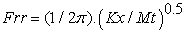(15)

is chosen at 8.5Hz in the range of the expected excitation frequency as previously calculated at § 3.3.1. The total mass is unchanged: 30 t. The Mt mass is thus elastically linked to the aircraft in the 0X direction. The code calculates acceleration, force, speed and displacements of the mass Mt along 0X.

Next Figure 10Figure 11 show that the motor modulation excites the suspended system which vibrates at its own resonance frequency of 8.5Hz.

The motor force Fm and the speed Vr are slightly modified with respect to the no-tank cases.

There are many parameters in the model that could modify the excitation frequency, notably the slip control strategy.

• Figure 10. K = 12.5: the motor thrust is modulated
• Figure 11. K = 12.5: Tank Vibrations

The resonance of the tank system is excited.

4.2. Case 4: Ls = 6.0 m, Ls= N = 12, Tank Resonance is not Excited (Figure 12 – Figure 13)

Next Figure 12-13 show that, with the ratio Ls/= N, a whole number, the vibrations are no more excited: There are small oscillations of the tank force at the beginning of the simulation due to the initial jerk of the jet engine being at full thrust. These oscillations are quickly damped. But during the rest of the launching the suspension is not excited (whatever the resonance frequency of suspended devices).

• Figure 12. K = 12: the motor thrust is not modulated
• Figure 13. K = 12: Smooth Tank acceleration, no resonance

### 5. Conclusions

In this paper we have presented a simplified numerical method calculating brake and motor forces of linear induction motors using a short metallic moving secondary in cooperation with a periodic magnetic field either sinusoidal or other.

The main result we have pointed to, that the length of the short secondary must be an exact multiple of the stator pole pitch, may seem obvious to some people. However we could not find such published statement.

Studying at first the simple braking case of a metallic sheet moving inside a stationary periodic magnetic field of constant wave length, we have demonstrated why the braking force is modulated with a wavelength equal to the inductor pole pitch. We also have demonstrated that these vibrations are always avoided when the ratio of the secondary length to the field pole pitch is a whole number.

Applying the brake arrangement analysis to the motor case, we have shown that the same unfavorable modulations always occur in the motor force when the ratio of the secondary length over the motor pole pitch is not a whole number. We have calculated the frequency of these modulations and shown how it depends on various parameters and notably the slip control strategy of the motor so that it can be difficult to avoid resonances of specific frequencies without sacrificing the search for the best motor efficiency which could require a different slip.

Building a dynamic model of the aircraft with suspended fuel tanks we have calculated resonance vibrations excited by the motor thrust. We propose that this modulation of linear induction motor forces can explain, at least partly, the vibrations observed during tests of the EMALS system.

The main result of this study is that the thrust or brake force of these linear induction motors arrangements is no more modulated as long as the length of the secondary sheet is an exact multiple of the stator pole pitch.

### Physical Parameters and Notations

A Maximum of B Field (sinus shape)

B Magnetic Field Flux Density B = [0 By 0]

Cx Suspension Damping

dF Force on dx Element

di Current through dx Element

dR Electric Resistance of dx Element

dx Length of numerical Secondary Element

Fx & Fm Force on Secondary Sheet (motor thrust)

Fj Permanent Thrust of the Jet Engine

Fm Target Value of Motor Thrust

Fra Frequency of Stator Current

Frm Frequency of the Force Modulation

Frr Mechanical Resonance Frequency

Hs Height of Secondary Sheet

K Ls/ratio, equals N when a Whole Number

Kx Suspension Stiffness

λ Field Wavelength, λLt Runway Length

Ls Length of Secondary Sheet

Mav Aircraft Mass

ρ Resistivity of the Secondary Sheet

s Motor SlipStator Pole Pitch

t Time

U & Uz Voltage Across dx Element

Vf Moving Field Velocity (fixed ref)

Vr Relative Velocity (Vr = Vf – Vs)

Vs & Vx Secondary Velocity (motor speed)

Ws Width of Secondary Sheet

### References

  Westinghouse ‘Electropult’, 1946, in: “A History of Linear Electric Motors”, E. R. Laithwaite, pp.46-47, Macmillan, London, 1987 In article  J. P. Pascal, «Rapport des essais du prototype du moteur linéaire à induit en U», Rapport de Recherche IRT, Paris, 1976, ed. IFSTTAR F 77447 Marne la Vallée, France https://filez.ifsttar.fr/jl3tds. In article  J. L. Giovachini, « Evaluation par la Similitude des Systèmes de propulsion et freinage électromagnétiques », Revue Générale des Chemins de Fer, Dunod, Paris, juin 1977. In article  EMALS, Kaman Electromagnetics corp., in: “Linear motor outperforms steam-piston catapults”, Automation & Motion Control, Oct. 23 1995 (www.designnews.com/automation-motion-control). In article  S. Gallagher, “Navy’s newest carrier has problems getting planes up”, ars Technica, 6/15/2017 (https://arstechnica.com/tech-policy/2017/06/uss-ford-is-ready-for-service-except-for-the-plane-launching-part/). In article  H. W. Beaty & J. L. Kirtley Jr., “Electric Motor Handbook”, Mc Graw Hill, NY, 1998. In article  G. Seguier & F. Notelet, « Electromagnétique Industrielle », 3rd ed. Lavoisier, Paris, 2006. In article  S. Yamamura, “Theory of linear induction Motors”, John Whiley & Sons, 1972. In article  E. R. Laithwaite, “Adapting a linear induction motor for the acceleration of large masses to high velocities”, IEE Proceedings, July 1995. In article View Article  A.H. Selcuk, Hasan Kurum, "Investigation of End Effects in Linear Induction Motors by Using the Finite-Element Method", IEEE Trans. on Magn., vol. 44, no. 7, pp. 1791-1795, Jul 2008. In article View Article  G. Kang, K. Nam, "Field-Oriented Control Scheme for Linear Induction Motor with the End-Effect", IEE Proc. on Elect. Pwr. Appl., vol. 152, no. 6, pp. 1565-1572, Nov 2005. In article View Article  A. Majumdar & T. K. Bhattacharya, “Field simulation of linear induction machines illustrating the peak to peak ripple in propulsive force and its dependence on the length of the primary”, 2017 International Conference on Power and Embedded Drive Control (ICPEDC), Chennai, India, Publisher: IEEE In article View Article  R. Feynman, “The Feynman Lectures on Physics”, California Institute of Technology, 2010 ed. (www.BasicFeynman.com). In article  A. Johnson, “High speed Linear Induction Motor Efficiency Optimization”, Dissertation Thesis, MIT, June 2005. In articleThis work is licensed under a Creative Commons Attribution 4.0 International License. To view a copy of this license, visit http://creativecommons.org/licenses/by/4.0/